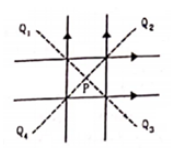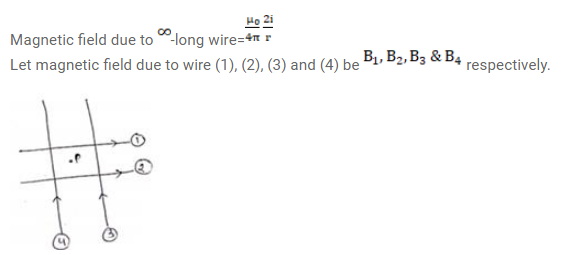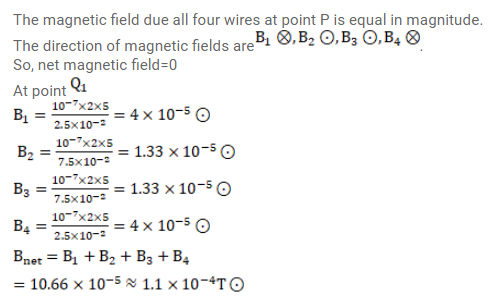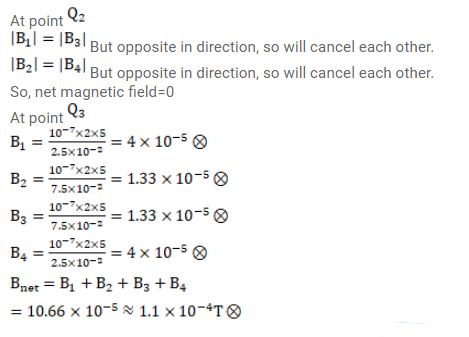# Four long, straight wires, each carrying a current of

Question:

Four long, straight wires, each carrying a current of $5.0 \mathrm{~A}$, are placed in a plane as shown in figure. The points of intersection form a square of side $5.0 \mathrm{~cm}$. (a) Find the magnetic field at the center $P$ of the square. (b) $\mathrm{Q}_{1}, \mathrm{Q}_{2}, \mathrm{Q}_{3}$ and $\mathrm{Q}_{4}$ are points situated on the diagonals of the square and at a distance from $P$ that is equal to the length of the diagonal of the square. Find the magnetic fields at these points.Solution: